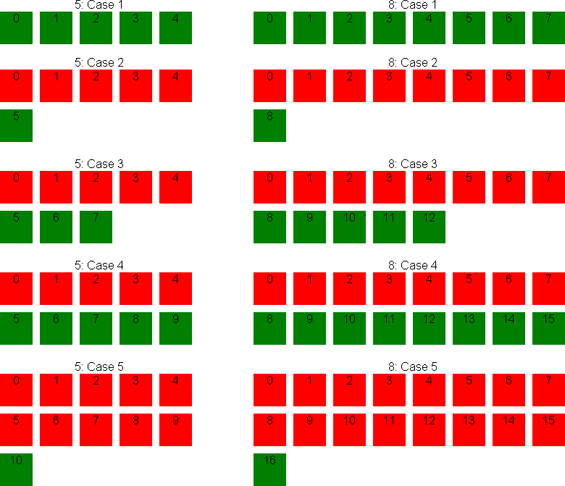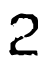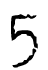# Determining if an element is in the last row of a tableOnce upon a time I stumbled upon a problem, where I needed to calculate if an element is in the last row of a table. Here's the scenario: you have a number of items, which are put in a table from left to right. When the row is full, the items continue in the next row. Imagine an airplane or a theater where people start sitting front-left and continue to the right until they run out of space, then going to the next row and so on. Now we want to know which people are sitting in the last of the populated rows.

A weird problem, but hopefully I will be able to show you some cool results produced by this algorithm someday (yes, it's usable).

The equation has 2 parameters: the total number of elements and the number of columns in a single row. There are a few ways to do it, using division with remainders (the modulo operation). The simple way would be comparing the total number of rows with the element's current row. Another one would be to calculate the number of elements in the last row and see if our element is in those last few. While both seem logically easy, they actually suck, because they contain exceptions (some states need to be handled specifically - if the last row is full or not).

That's why I went for the ultimate way, using abstract mathematics which requires real magic, and since it would be too boring to explain it, just take it if you need it and don't try to understand - to be honest, even I'm not perfectly sure how I did it.

## The number of rows way:

lastRow = ((Math.DivRem(currentElementIndex + 1, numberOfColumns, out remainder1) + Convert.ToInt16(remainder1 > 0)) >= Math.DivRem(totalElements, numberOfColumns, out remainder2) + Convert.ToInt16(remainder2 > 0))
* Math.DivRem returns the division result and the remainder, since both are required for the calculation

## The number of items in the last row way:

lastRow = (totalElements - (totalElements % numberOfColumns) - (numberOfColumns * Convert.ToInt16((totalElements % numberOfColumns) == 0)) < currentElementIndex + 1)
* % is the modulo - remainder from dividing

## The ultimate way:

lastRow = totalElements < ((numberOfColumns + currentElementIndex) - (currentElementIndex % numberOfColumns) + 1)
* % is the modulo - remainder from dividing

Mathematics is awesome.

UPDATE (27.7.2011): Silly me. Overwhelmed by "The ultimate way", I missed the opportunity to simplify "The number of rows way". I guess this is one of the cases which explain why counting starts with 0 instead of 1 in programming.

## The improved number of rows way:

lastRow = Math.DivRem(currentElementIndex, numberOfColumns, out result1) >= Math.DivRem(totalElements - 1, numberOfColumns, out result2))
* Math.DivRem returns the division result and the remainder, since we need the number rounded down.Categories: Chronolog, Data, Design, Lifehacks, Mathematics, Software, Webdev
Like what you read? Now tell the world!
 Tweet Vote on Hacker News

A few more things you might find interesting:

Comment
written 25.7.2011 12:57 CET on chronolog
1961 views   •   1 like   •   Like   •Connect with :